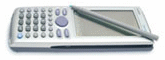# How To Divide Polynomials ?

### #1MithrandiR

MithrandiR

Newbie

•• Members
•• 9 posts

• Calculators:
CP 300

Posted 07 November 2004 - 04:39 AM

it works when i divide trinomial by a binomial but it doesnt seem to work when i divide polynomial with 4 monomials by a binomial

example:
(x^3+8x^2-20x)/(x-2)
this one works well

(2x^3-3x^2+3x-4)/(x-2)
this one doesnt seem to work

(x^5+32)/(x+2)
doesnt seem to work as well

any1 plz help me

and any1 plz xplain the different btween command "factor" and "rFactor", "expand" and "tExpand", "solve", "dSolve" and "rSolve"

thx !

### #2Lovecasio

Lovecasio

Casio Freak

•• Members
•• 242 posts
• Location:Hochiminh city Vietnam
• Interests:Organic chemistry.<br />Pharmacy

• Calculators:
fx 570 MS, Casio AFX 2.0+, ClassPad 300

Posted 07 November 2004 - 05:59 AM

Hi.To divide a polynomial, the best function is propFrac ( in Action/Transformation ). This functions will suit your needs. ( I guess that you use simplify func, right? It just works when there is no remainder in calculation ).
-factor: factor an expression.
-rFactor : can be simply though to factor as much as possible.
-expand: syntax: expand(exp) => normal expandation or expand(exp,var) => special expand. Ex: expand(1/((x+1)(x-1)),x) => 1/(2(x-1))-1/(2(x+1)).
-solve, dSolve and rSolve : solving equation or inequation, solving differential equation, solve sequence formula.
-tExpand: trigonometric expand.

Read user manual for more information.• sascha likes this

#### 0 user(s) are reading this topic

0 members, 0 guests, 0 anonymous users